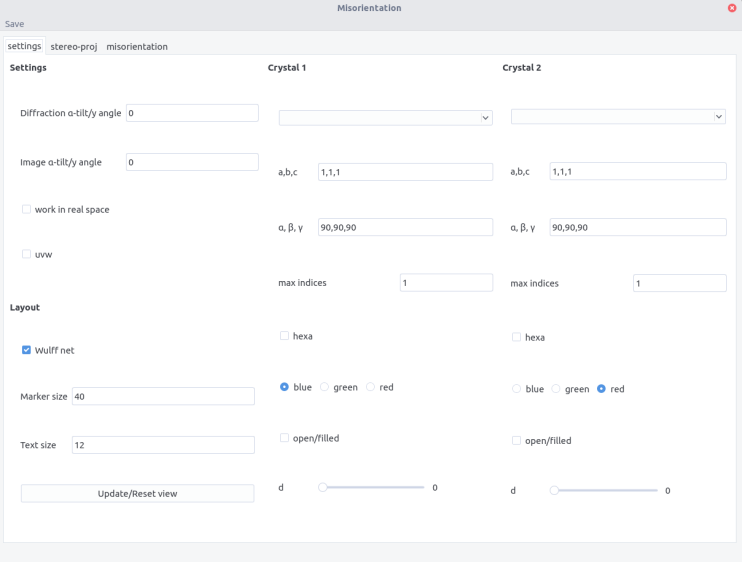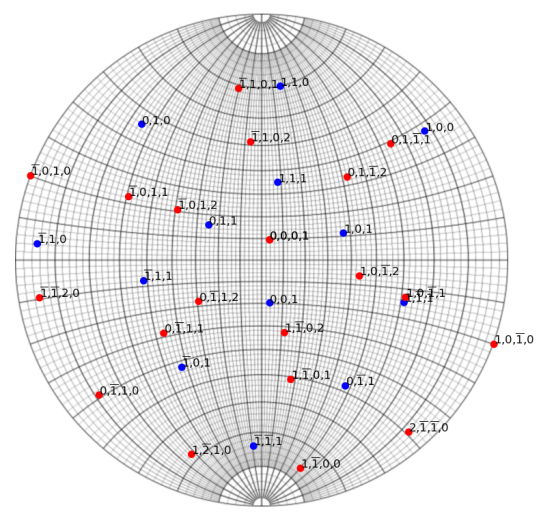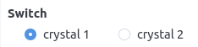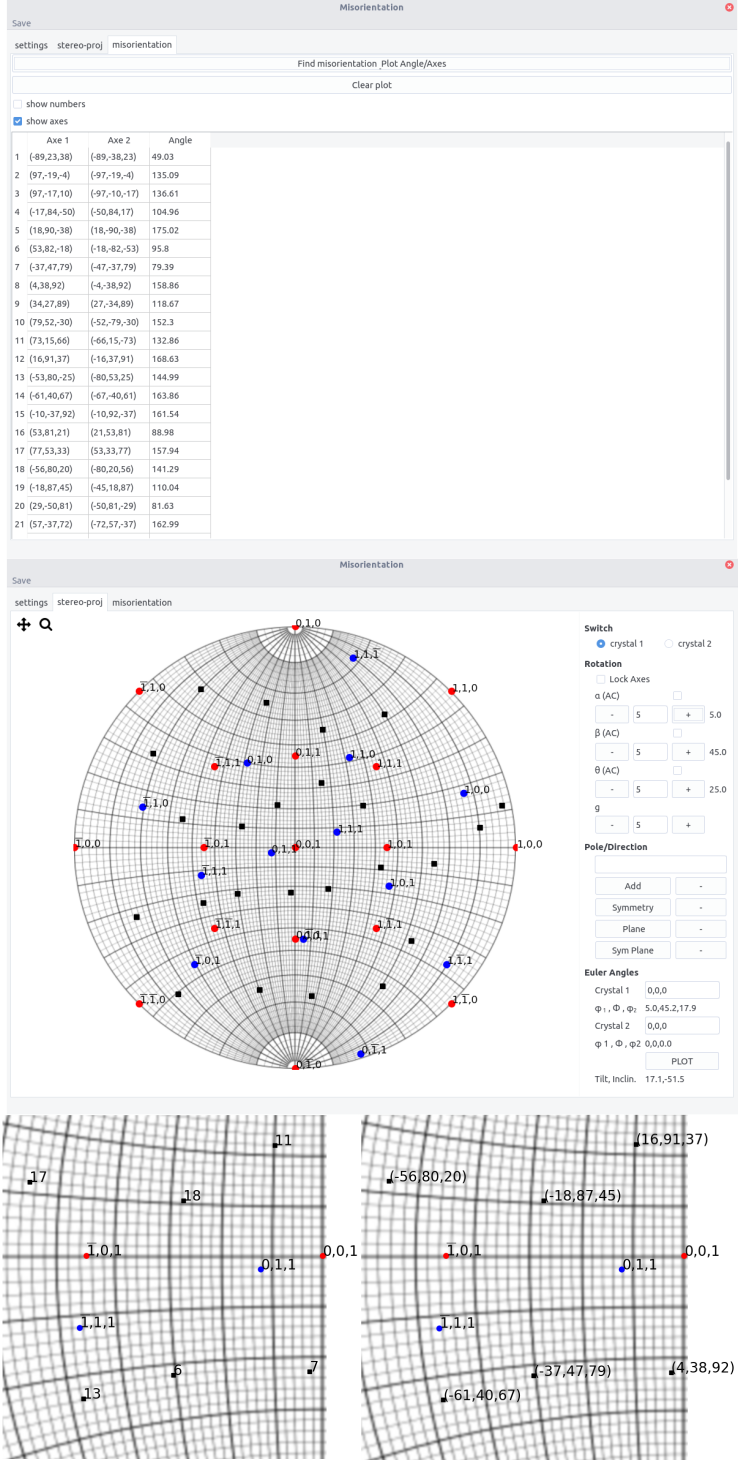# Misorientation

misorientation allows to determine orientation relationship and misorientation between two crystals It allows:

• Display two crystals at the same time on a stereographic projection which allows the easy determination of orientation relationship

• Orient two crystals independently

• Determine misorientation between two grains (same crystals)

## Misorientation¶

When two crystals are separated by a grain boundary, the neighboring crystals may exhibit a specific orientation relationship that can be defined by an interface and a couple rotation axis, i.e. a common crystal direction, misorientation angle.

Considering the orientation of grains $A$ and $B$ defined by their Euler angles. Their orientation in the reference frame is defined by the orientation matrix $O_1$ and $O_2$. The misorientation matrix is given by:

M=O_1 O_2^{-1}

As $M$ is a rotation matrix, one could extract the couple angle-axis, $(\theta,\vec{u})$ according to:

\theta=\arccos \left(\frac{tr(M)-1}{2}\right)

and

M\vec{u}=\vec{u}

However, because of crystal symmetry, the misorientation between two grains is not unique, i.e. equivalent $(\vec{u},\theta)$ exist. The misorientation axis should then be modified by:

M=O_1O_SO_2^{-1}

where $O_S$ are the symmetry operations of the crystal. For cubic, it consists of:

• Rotations along $\langle 100 \rangle$ of 90°, 180° and 270° (9 rotations)

• Rotations along $\langle 110 \rangle$ of 180° (6 rotations)

• Rotations along $\langle 111 \rangle$ of 120° and 240° (8 rotations)

• Identity operation

Thus 24 symmetry operations can be applied, leading to 24 $(\vec{u},\theta)$ couples.

Equivalent considerations can be made for other crystal systems.

## Interface¶

The interface is composed of 3 tabs.

• the settings tab is used to enter the 2 crystal inputs, the microscopic settings regarding rotation between tilt axis and image, and the layout. Refer to stereoproj for the details information.

• the stereo-proj tab is the plotting area

• misorientation tab display the misorientation between crystal (if identical crystal are selected)## Procedure¶

### Plotting¶

• Set the crystal parameter and layout in the setting tab. Refer to stereoproj for the details.

• Enter the crystal 1 and 2 orientation in the Euler Angles fields

• Plot the stereographic projections using the Plot buttonOverlay of an aluminium and an zirconium crystal.

• Same additional features as in stereoproj are available: adding poles/directions, planes... and can be performed independently on crystal 1 or 2 by using the Switch button• Rotations can be performed along $x,y,z$ or along a pole/direction ($g$) entered in the Pole/direction field, either in crystal 1 or 2

### Misorientation¶

For 2 crystals of the same structure, the misorientation can be computed after plotting the stereographic projection.

In the misorientation tab:

• press the Find misorientation Plot Angle/Axis button

• the rotation axes in both crystal are displayed along with the rotation angle

• if the uvw button is ticked in the setting tab, the axes will be given as directions and not plane normals.

• in the stereographic tab, the axes appear as black dots.

• ticking the show numbers or show axes will display the number corresponding to the angle/axis couples and the axis indices in crystal 1 on the stereographic projection, respectively.